Ex 15.1

Chapter 15 Class 8 Introduction to Graphs
Serial order wise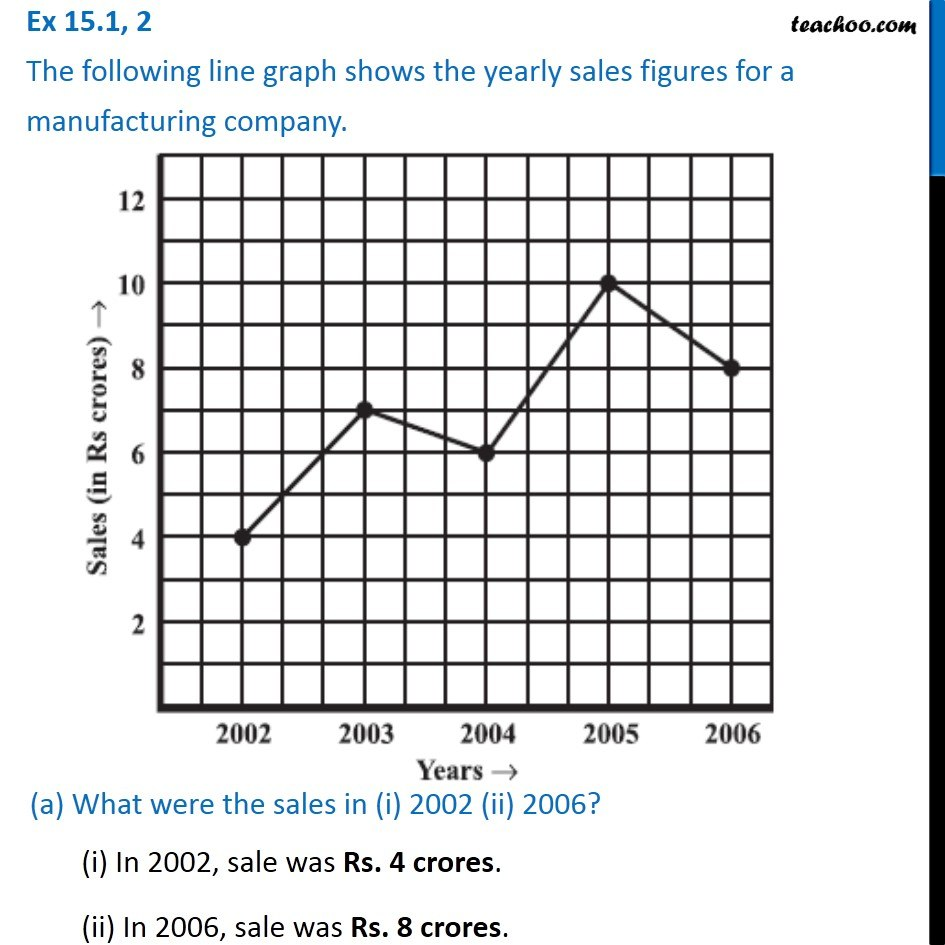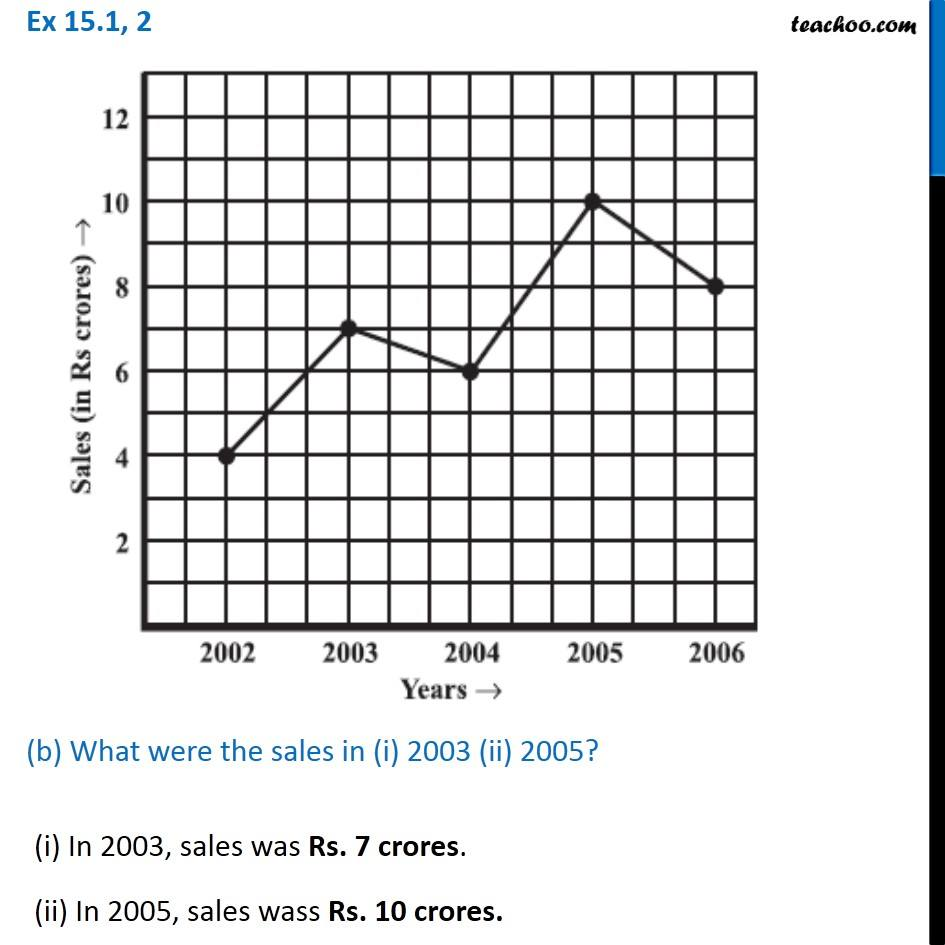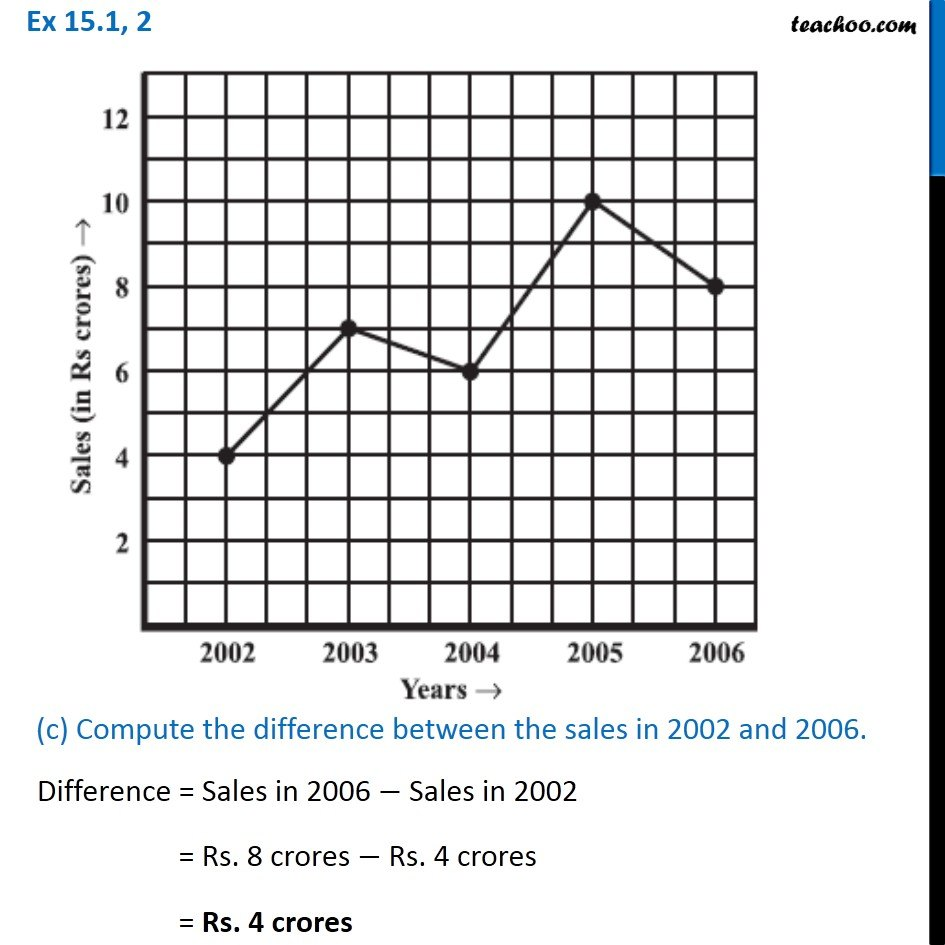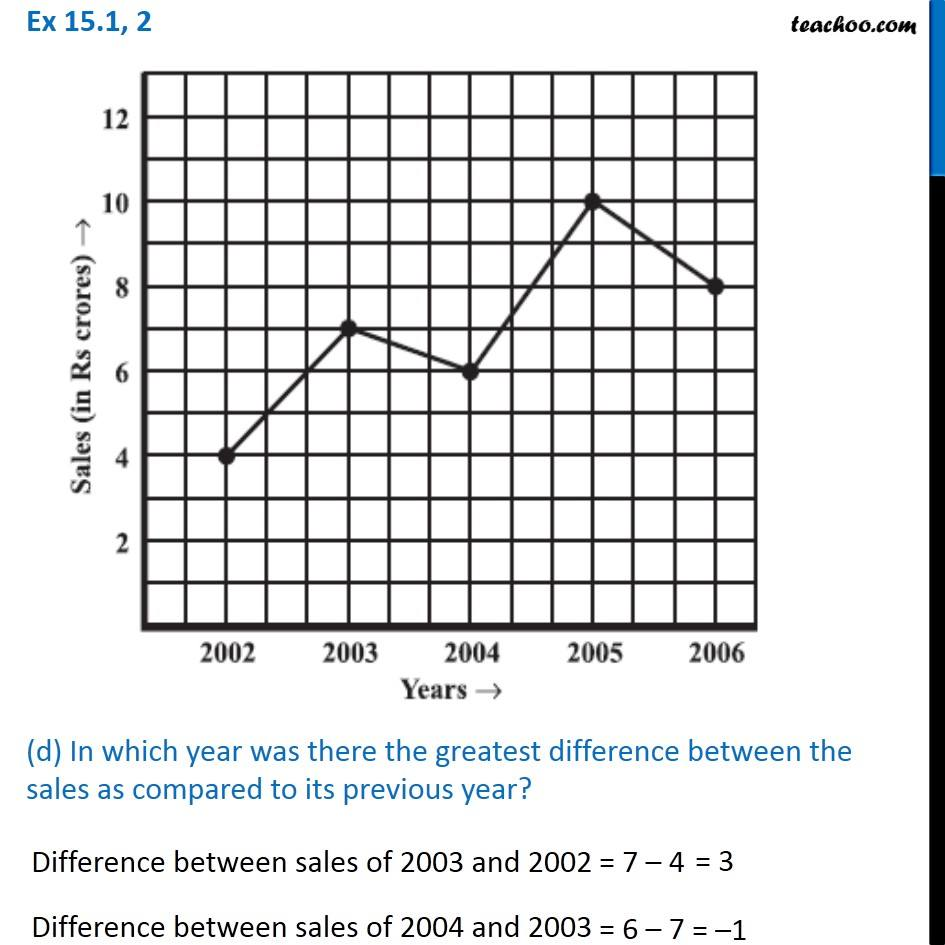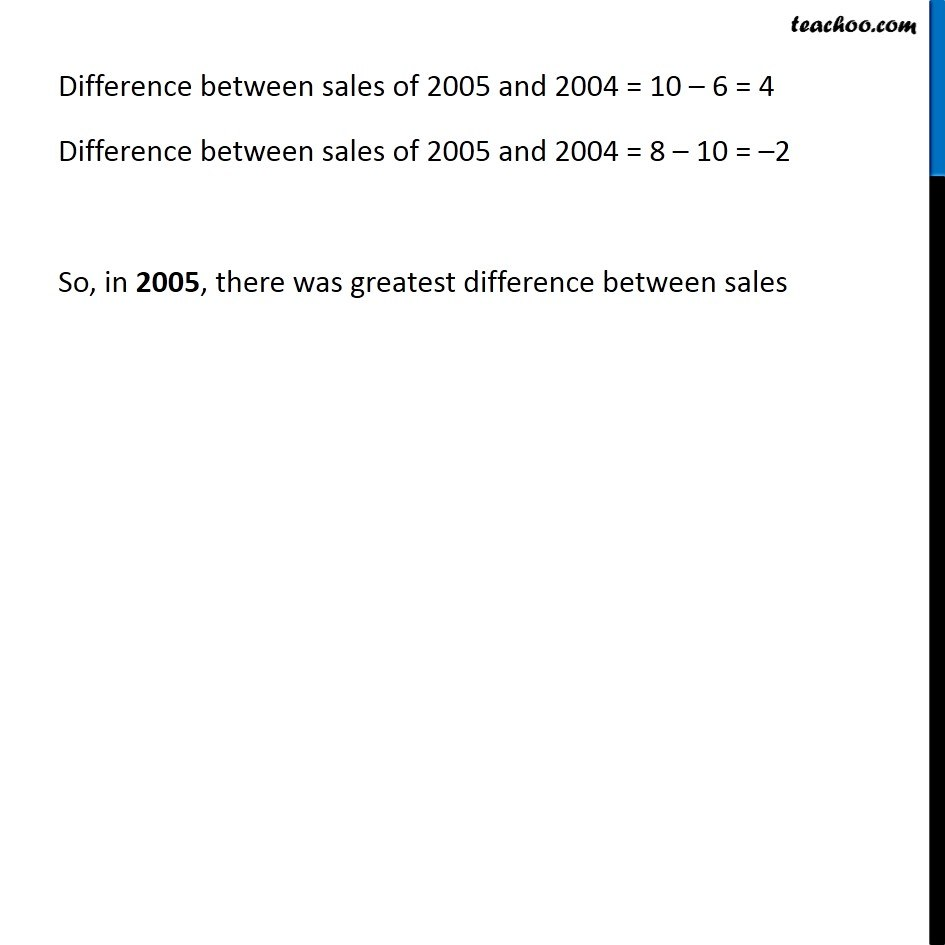### Transcript

Ex 15.1, 2 The following line graph shows the yearly sales figures for a manufacturing company. (a) What were the sales in (i) 2002 (ii) 2006? (i) In 2002, sale was Rs. 4 crores. (ii) In 2006, sale was Rs. 8 crores. (b) What were the sales in (i) 2003 (ii) 2005? (i) In 2003, sales was Rs. 7 crores. (ii) In 2005, sales wass Rs. 10 crores. (c) Compute the difference between the sales in 2002 and 2006. Difference = Sales in 2006 − Sales in 2002 = Rs. 8 crores − Rs. 4 crores = Rs. 4 crores (d) In which year was there the greatest difference between the sales as compared to its previous year? Difference between sales of 2003 and 2002 Difference between sales of 2004 and 2003 Difference between sales of 2005 and 2004 = 10 – 6 = 4 Difference between sales of 2005 and 2004 = 8 – 10 = –2 So, in 2005, there was greatest difference between sales